Next: Perturbation Expansion Up: Time-Dependent Perturbation Theory Previous: Two-State System

# Spin Magnetic Resonance

Consider a system consisting of a spin one-half particle with no orbital angular momentum (e.g., a bound electron) placed in a uniform-directed magnetic field, and then subject to a small time-dependent magnetic field rotating in the-plane at the angular frequency. Thus,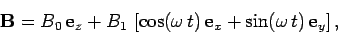(1045)

whereand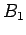are constants, with. The rotating magnetic field usually represents the magnetic component of an electromagnetic wave propagating along the-axis. In this system, the electric component of the wave has no effect. The Hamiltonian is written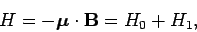(1046)

where(1047)

and(1048)

Here,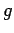andare the gyromagnetic ratio [see Eq. (1004)] and mass of the particle in question, respectively.

The eigenstates of the unperturbed Hamiltonian are the spin up'' and spin down'' states, denoted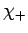and, respectively. Of course, these states are the eigenstates ofcorresponding to the eigenvalues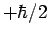and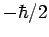respectively (see Sect. 10). Thus, we have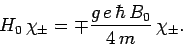(1049)

The time-dependent Hamiltonian can be written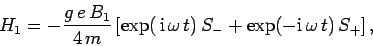(1050)

whereandare the conventional raising and lowering operators for spin angular momentum (see Sect. 10). It follows that(1051)

and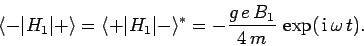(1052)

It can be seen that this system is exactly the same as the two-state system discussed in the previous subsection, provided that the make the following indentifications: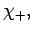(1053)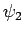(1054)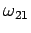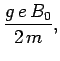(1055)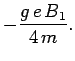(1056)

The resonant frequency,, is simply the spin precession frequency in a uniform magnetic field of strength(see Sect. 10.6). In the absence of the perturbation, the expectation values ofand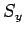oscillate because of the spin precession, but the expectation value ofremains invariant. If we now apply a magnetic perturbation rotating at the resonant frequency then, according to the analysis of the previous subsection, the system undergoes a succession of spin flips,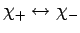, in addition to the spin precession. We also know that if the oscillation frequency of the applied field is very different from the resonant frequency then there is virtually zero probability of the field triggering a spin flip. The width of the resonance (in frequency) is determined by the strength of the oscillating magnetic perturbation. Experimentalists are able to measure the gyromagnetic ratios of spin one-half particles to a high degree of accuracy by placing the particles in a uniform magnetic field of known strength, and then subjecting them to an oscillating magnetic field whose frequency is gradually scanned. By determining the resonant frequency (i.e., the frequency at which the particles absorb energy from the oscillating field), it is possible to determine the gyromagnetic ratio (assuming that the mass is known).Next: Perturbation Expansion Up: Time-Dependent Perturbation Theory Previous: Two-State System
Richard Fitzpatrick 2010-07-20